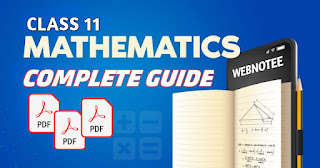Every student studying in Grade 11 needs help when solving mathematics questions. You are not the only one who faces difficulties while solving mathematics questions. When we get stuck on certain questions, we need a mathematics guide. To be more precise, we need a Class 11 Mathematics solution PDF.

When you want the Class 11 Basic Mathematics solution PDF, you search here and there. In the end, you won't find any helpful notes or guides. When I was studying in Grade 11, I used to face these problems a lot. I would spend hours and hours searching for the notes on Google but found none.

I think I'm not the only one who searches for notes online. I wanted to make sure that you don't have to face the problems I have faced before. In order to help you out, I have brought a complete solution PDF for Class 11 Basic Mathematics. Scroll down to find the download link for the PDF.

##In class 11 we study Basic Mathematics and most of the school uses the book by Sukunda Pustak Vawan. It is considered as one of the suitable book for grade 11 as it contains exercises, MCQs as well as additional questions which can help students to score good marks in mathematics

Inorder to help you navigate the chapters, I have added the table of content below along with the chapters name. Just click on the 'Get Notes' button to get the  notes of the respective chapter.

Note: We may not have the solutions of some chapters. If you have then you can submit us through Gmail.

#### I. Algebra

Chapter 1: Logic, Sets and Real Number System

Chapter 2: Functions

Chapter 3: Sketching

Chapter 4: Sequence and Series

Chapter 5: Metrics and Determinants

Chapter 7: Complex Numbers

#### II. Trigonometry

Chapter 8: Inverse Circular Functions and Trigonometric Equation

#### III. Analytic Geometry

Chapter 9: Analytical Geometry

Chapter 10: Pair of Straight Lines

Chapter 11: Coordinates in Space

#### IV. Vectors

Chapter 12: Vectors

#### V. Statistics and Probability

Chapter 13: Measures of Dispersion

Chapter 14: Probability

#### VI. Calculus

Chapter 15: Limits and Continuity

Chapter 16: The Derivatives

Chapter 17: Application of Derivatives

Chapter 18: Antiderivatives

#### VII. Computational Methods

Chapter 19: Numerical Computation

Chapter 20: Numerical Integration

#### Mechanics

Chapter 21: Statics

## Why Class 11 Mathematics Solutions Matter?

Class 11 Mathematics can be challenging for students and having access to reliable solutions is very important for students. Solutions can play a vital role by providing step-by-step explanations on how to solve mathematical problems. They serve as helpful guides which can help you in understanding the concepts and techniques involved.

The importance of class 11 mathematics solutions goes beyond simply obtaining correct answers. It can help students by enhancing their overall knowledge of the respective subject. Furthermore, these solutions serve as valuable resources for students, equipping them with strategies to tackle similar problems in the future.

With the help of class 11 mathematics solution, you can easily solve any mathematics problems of any chapter of  gade 11. I'm telling that because it contains the notes of almost all chapters that you have to study in grade 11.

## Strategies for Effective Use of Class 11 Mathematics Solution PDFs

Class 11 mathematics solution PDFs contains all questions solutions of the entire chapter taught in grade 11. But, you shouldn't just copy each and every solution from the PDF. If you do that then you will get weaker in mathematics day by day. I strongly recommend you to use this PDFs wisely. Don't overuse it because it has negative sides too.

You should look at the PDFs solutions only when you get stuck while solving the questions given in your exercise. Just look the process of solving the specific questions and close the PDF and try solving that question yourself. If you use the PDFs in right way then it will be beneficial for you.

At last I want to suggest you to give your 100% best while solving mathematics questions. It is because if you solve the questions yourself then you won't forget it for a long time. So at least try 2-3 times before looking at the PDF for the solution of the questions.

## FAQs

1. Which is the best guide for maths class 11?

If you are searching for the best guide for class 11 maths then you need to buy the solution set for grade 11 basic mathematics. If you don't want to waste your money then you can download our mathematics guide PDF from the link given above. We have the solutions for each and every chapters.

2. What are the topics in math for 11?

There are many topics that we need to study in grade 11 mathematics. Among them derivatives and antiderivatives covers the most of the parts. It is among the lengthy chapter of grade 11 mathematics.

3. How many chapters are there in mathematics class 11?

In grade 11 mathematics, there are 21 chapters in total and among them one is optional. In most of the cases we don't study mechanics portion as it falls under optional part.

If these solutions are helpful to you then feel free to share it with your friends and classmates 😊
•